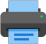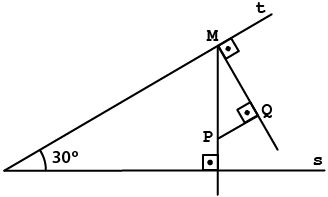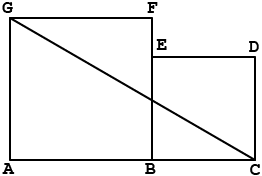Lista de exercícios do ensino médio para impressãoSelect all.
print selected exercises
(FUVEST - 1977)
Given that:
$\,\overline{MP}\;\bot\;s\,$;$\;\overline{MQ}\;\bot\;t\,$;$\;\overline{MQ}\;\bot\;\overline{PQ}\,$;$\;\overline{MP}\,=\,6$
The length of $\,\overline{PQ}\,$ é is:
a)
$\,3\sqrt{3}\,$
b)
$\,3\,$
c)
$\,6\sqrt{3}\,$
d)
$\,4\sqrt{3}\,$
e)
$\,2\sqrt{3}\,$answer: (B)
×
In the picture below, ABFG and BCDE are squares and their each one sides measures, respectively, a and b. Knowing that $\;\overline{AG}\,=\,\overline{CD}\,+\,2\;\,$ and the perimeter of the triangle ACG is 12, we can assure that a e b are both inside the interval:
a)
]1; 5[
b)
]0; 4[
c)
]2; 6[
d)
]3; 7[
e)
]4; 8[answer: (B)
×
Other math tests:
similar triangles
triangles similarity